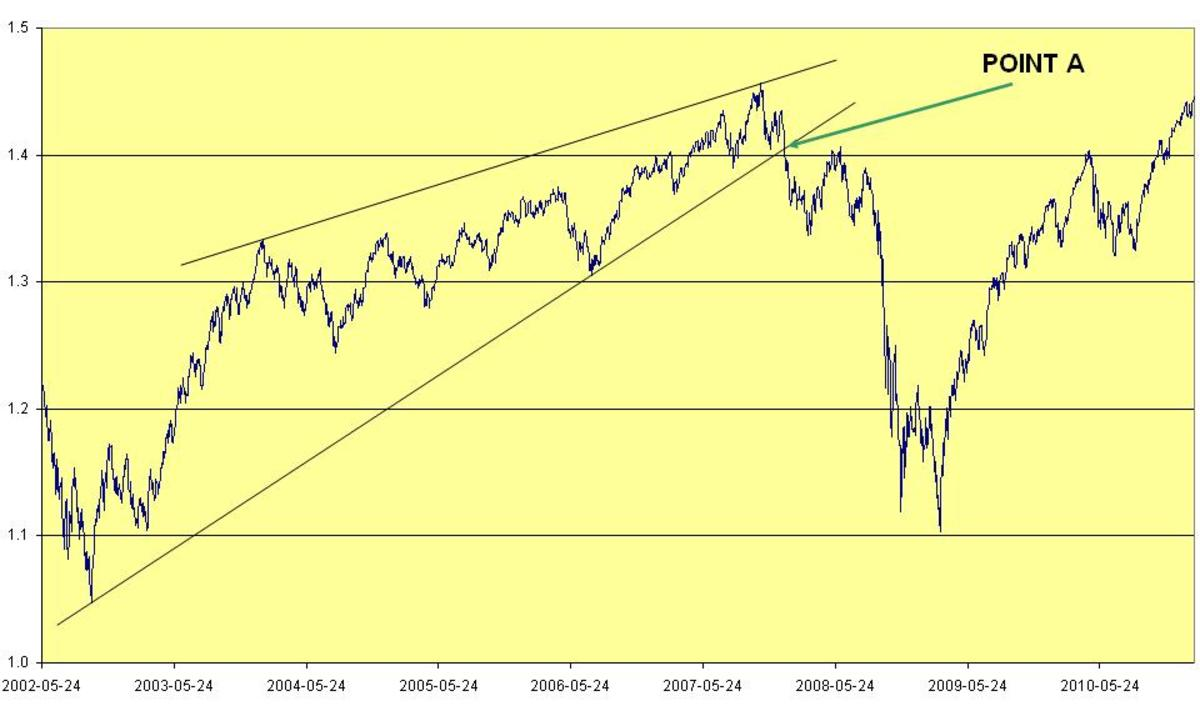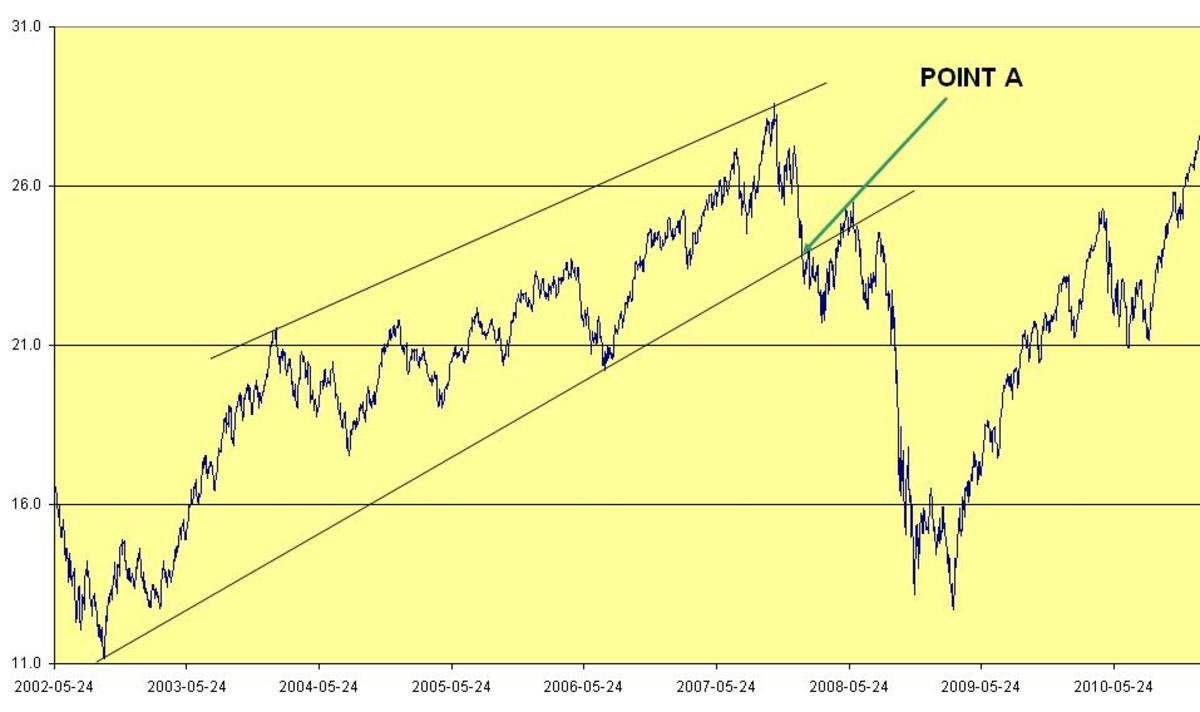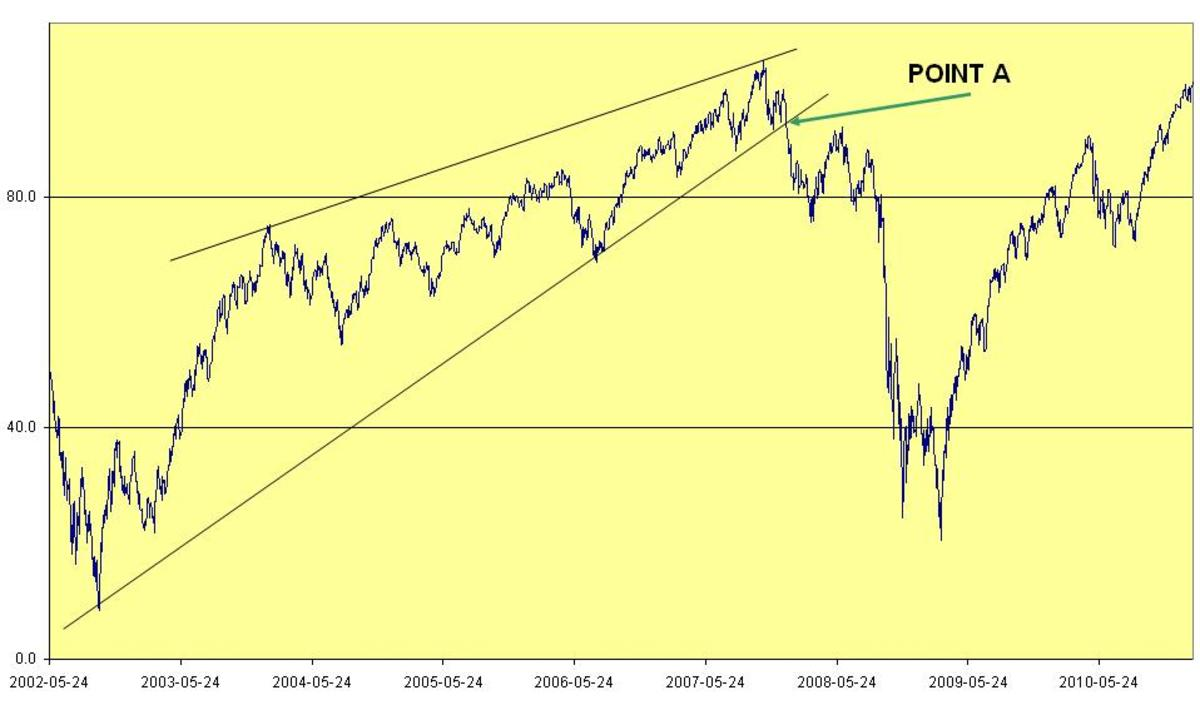# How to Trade Stocks - Plot Semi Log Graph in Excel – and Logarithmic Scale in Excel

• Author:
• Updated date:

## A Picture Is Worth a Thousand Words

In real life, a picture is worth a thousand words. This is also true in science and stock market. A simple image or a chart can easily explain complex ideas that would otherwise have been difficult to visualize. In science, when you deal with a wide range of data values and you plot them on a graph to establish a relationship with another set of data of a restricted range, you get a graph that hides some features from your eyes. To be able to see the hidden features realistically, the scientist need plot the data values using a logarithmic scale. This is also true in the analysis of stock prices.

Example: You buy the stock CSCO at \$10 and after one month the price is \$11, an increase of \$1.00. At the same time, your spouse buy the IBM stock at \$100 and after one month the price is \$110, an increase of \$10.00.  Naturally, it’s possible to start thinking that your spouse has done well in the stock trade than yourself. Logically, the two trades are the same on a logarithmic scale but completely different on a linear scale.

## Plotting a Semi Log Graph in MS Excel

If you have MS excel in your computer, then, plotting a semi log graph in Excel is quit easy and straight forward. All you do is this:

1. Select the data (wide data range) you want to plot and then select insert chart
2. Select the type of chart (line) and then finish
3.  Right click on data value axis and then format Axis
4. Select scale and then check Logarithmic Scale and you are done
5. You axis will now have new data values ranging greater than zero up to 100.

So, what do the data values ranging greater than zero up to 100 represent? If you have to make any good value of your Semi Log Graph, then there is need for you to understand what the data values ‘ranging greater than zero up to 100’, on your axis, represent.

## Logarithm

In mathematics,

1.  If x = 10ª, then a = Log10[x]

Log  =Log [10³]

Log [10³] = 3 Log 

Log  = 1, therefore, 3 Log  = 3

Therefore, Log  = 3

2.  Log  = Log [10²]

Log [10²] = 2 Log 

Log  = 1, therefore, 2 Log  = 2

Therefore, Log  = 2

On linear scale, a plot of 100 and 1000 would correspond to 2 and 3 on Logarithmic Scale respectively.

## Plot Semi Log Graph using Excel Formulas:

In this first example on how to Plot Semi Log Graph using Excel Formulas, we use daily closing prices on NASDAQ Composite for the last nine years, or so. That is wide range of data.

1. Get free NASDAQ Composite data from Yahoo Finance
2. In excel spreadsheet, copy and paste the corresponding dates and NASDAQ closing prices in column A and column B respectively.
3. In column C, insert the formula, “=Log(B1)”
4. Drag down the formula in 2 above in as much as your data goes.
5. Select using control key the data in column A and column C
6. Select insert chart, select the type of chart (line) and then finish
7. You are done.

You should get a Semi Log Graph in Excel as shown in the chart below.

We had stated earlier that, Log  = 2

Scroll to Continue

This means that antilog of 2 = 100

From the vertical axis in your graph, you will need to get the antilog of the values shown on the axis so that the antilog will be the price of the stock/security in dollars.Plot Semi Log Graph using Excel Formulas

## Plot Linear Graph using Excel Formulas

In this second example on how to Plot Linear Graph using Excel Formulas, we use daily closing prices on NASDAQ Composite for the last nine years or so. That is a lot of data.

1. Get free NASDAQ Composite data from Yahoo Finance

2. In excel spreadsheet, copy and paste the corresponding dates and NASDAQ closing prices in column A and column B respectively.

3. Select using the control key the data in column A and column B

4. select insert chart, select the type of chart (line) and then finish

5. You are done.

You should get a Linear Graph in Excel as shown in the chart below.

From the vertical axis in your graph, what is shown are the actual prices of the stock/security in dollars (in my case, I had initially divided the NASDAQ Composite prices by 100 before plotting the graph because I like working with numbers of few digits).Plot Linear Graph using Excel Formulas

## Percentage Terms

When people talk in percentage terms, it’s not always easy to understand for many people. Look at this example:

1. If the price of stock advance from \$100 to \$200, we say it’s an increase of ((200-100)/100)*100% = 100% increase.

2. If the price of the same stock then falls from \$200 to \$100, we say it’s a decrease of ((200-100)/200)*100% = 50% decrease.

So, price change from \$100 to \$200 is 100% and price change from \$200 to \$100 is 50%. Why this huge difference of 50% and 100% when what happened today is just the ‘opposite’ of what happened yesterday. Do you think the common person can be able to visualize this?

## Plot Cumulative Percentages Change Graph Using Excel Formulas

In this third example on how to Plot Cumulative Percentages Change Graph using Excel Formulas, we use daily closing prices on NASDAQ Composite for the last nine years or so. That is a lot of data which has a wider range.

1. Get free NASDAQ Composite data from Yahoo Finance

2. In excel spreadsheet, copy and paste the corresponding dates and NASDAQ closing prices in column A and column B respectively.

3. Borrowing from percentages terms above, insert in column C1 the formula, “=IF(B1>B2,-100*(B1-B2)/B2,100*(B2-B1)/B1)”

4. Insert “100” in Column D, row 1

5. insert in column D, row 2, the formula, “=D1+C1

6. Drag down the formula in column D, row 2, in as much as your stock data goes.

7. Select using the control key the data in column A and column D

8. select insert chart, select the type of chart (line) and then finish

9. You are done.

You should get Cumulative Percentages Change Graph in Excel as shown below.

Note that the first (current) value in the y-axis is always 100. You will note that any time you add new data values, the values on the entire data points in the graph changes. This means new trend lines have to be plotted afresh every day that you get new stock data values.Plot Cumulative Percentages Change Graph Using Excel Formulas

## Trend Lines

Trend lines are drawn for analyzing price charts and are widely used in trading stocks, futures, commodities and currencies. Whenever the price line bounces on a trend line, it signifies continuation of the trend. When price line breaks the trend line, it signifies trend reversal. In other words, trend lines are taken to be the point of support and resistance.

We can therefore say that trend line in a price charts indicates:

1. Direction of the current price movement.

2. The strength/gradient of price movement

3. Probable future points of support and resistance of price movement

## Comparing the Three Charts

If you look at the three charts above, you will notice that the Semi Log Graph is very similar to the Percentages Change Graph. By looking at the trend lines drawn on the graphs, one can see that the prices are converging. This feature is completely hidden in the Linear Graph. Assuming one trader T1 was using the Semi Log Graph and trader T2 was using the linear graph, trader T2 would have failed to notice the convergence developing and would thus have been in more difficulties in predicting future price movement when the price line moved past point A (shown in all the graphs).

## A Good Stock Trading Strategy for a Beginner Trader

We can conclude and say that it does not matter if you use linear graph or Semi Log Graph for trading short term. If you are trading for a long term, say more than 2 years, then, it is very important you use Cumulative Percentages Change Graphs or Semi Log Graphs. Use of trend lines can be a good Stock Trading Strategy for a beginner trader if the art of plotting the trend lines on semi log graphs and linear graphs is well mastered.

If you have liked this article, and you would want this page to keep up and improved, you can help by purchasing some great items from Amazon by following Amazon links and widgets on this page. A free way to help would be to link back to this webpage from your web page, blog, or discussion forums.

The Author’s page is designed to help beginners and average readers make some money as an extra income to supplement what they may be earning elsewhere - details of which you can find in My Page.

monicamelendez from Salt Lake City on July 26, 2012:

I don't know how I ended up on this hub but dang...you're a lot smarter than I am. ;)

Jon Peterz from California on September 24, 2011:

Great informative post.

I want to try this out.

Thanks.

Share Trading on August 07, 2011:

Fantastic and expert article. Very nicely and clearly explained. All newbies to share trading must read this.

forexprophecy on April 08, 2011:

This is something I have seen for first time.Good Job Sir!

chamilj from Sri Lanka on April 04, 2011:

Excellent explanation about Stock Charts. Thanks for your good work!

KennyG-not him-Me from Louisville, KY on March 28, 2011:

Very informative. Thanks for the information.

Hello, hello, from London, UK on February 11, 2011:

Thank you for an interesting and very detailed hub.